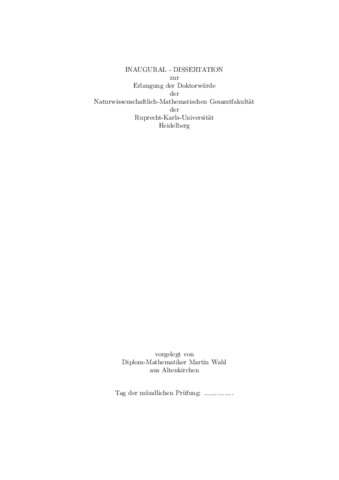On the Mod-Gaussian Convergence of a Sum over Primes and Estimation of Components and Variable Selection in High-Dimensional Complex Models

Wahl, MartinPreview
PDF, English - main document
Download (811kB) | Lizenz: Print on Demand

Citation of documents: Please do not cite the URL that is displayed in your browser location input, instead use the DOI, URN or the persistent URL below, as we can guarantee their long-time accessibility.

Abstract

This thesis considers two problems, one in probabilistic number theory and another in mathematical statistics.

In Chapter 1, we study the distribution of values taken by the logarithm of the Riemann zeta-function on the critical line. We prove mod-Gaussian convergence for a Dirichlet polynomial which approximates $\operatorname{Im}\log\zeta(1/2+it)$. This Dirichlet polynomial is sufficiently long to deduce Selberg's central limit theorem with an explicit error term. Moreover, assuming the Riemann hypothesis, we apply the theory of the Riemann zeta-function to extend this mod-Gaussian convergence to the complex plane. From this and the theory of large deviations, we obtain that $\operatorname{Im}\log\zeta(1/2+it)$ satisfies a large deviation principle on the critical line. Results about the moments of the Riemann zeta-function follow.

In Chapter 2, we consider the nonparametric random regression model $Y=f_1(X_1)+f_2(X_2)+\epsilon$ and address the problem of estimating the function $f_1$. The term $f_2(X_2)$ is regarded as a nuisance term which can be considerably more complex than $f_1(X_1)$. Under minimal assumptions, we prove several nonasymptotic $L^2(\mathbb{P}^X)$-risk bounds for our estimators of $f_1$. Our approach is geometric and based on considerations in Hilbert spaces. It shows that the performance of our estimators is closely related to geometric quantities from the theory of Hilbert spaces, such as minimal angles and Hilbert-Schmidt norms. Our results establish general conditions under which the estimators of $f_1$ have up to first order the same sharp upper bound as the corresponding estimators of $f_1$ in the model $Y=f_1(X_1)+\epsilon$. As an example we apply the results to an additive model in which the number of components is very large or in which the nuisance components are considerably less smooth than $f_1$.

In Chapter 3 and 4, we consider the problem of variable selection in high-dimensional sparse additive models. We focus on the case that the components belong to nonparametric classes of functions. The proposed method consists of comparing the norms of the projections of the data onto various additive subspaces. Under minimal geometric assumptions, we prove concentration inequalities which lead to general conditions under which consistent variable selection is possible. Again, our approach is based on geometric considerations in Hilbert spaces. Moreover, we apply recent techniques from the theory of structured random matrices to accomplish the transition from the $L^2(\mathbb{P}^X)$-norm to the empirical norm. As an application, we establish conditions under which a single component can be estimated with the rate of convergence corresponding to the situation in which the other components are known. Finally, we derive optimal conditions for variable selection in the related and more simple additive Gaussian white noise model.

Item Type: Dissertation Mammen, Prof. Dr. Enno 13 April 2015 20 Apr 2015 11:20 2015 The Faculty of Mathematics and Computer Science > Department of Applied Mathematics 510 Mathematics